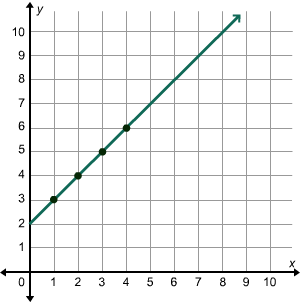# Generating Patterns and Graphing Relationships

Rate 0 stars Common Core
Quiz size:
Message preview:
Someone you know has shared Generating Patterns and Graphing Relationships quiz with you:

To play this quiz, click on the link below:

https://www.turtlediary.com/quiz/generating-patterns-and-graphing-relationships.html

Hope you have a good experience with this site and recommend to your friends too.

Login to rate activities and track progress.
Login to rate activities and track progress.

Consider the table given below.

 x y 1 3 2 4 3 5 4 6

Let's find the relationship between x and y.

Observe that:

Row 1: 1 + 2 = 3

Row 2: 2 + 2 = 4

Row 3: 3 + 2 = 5

Row 4: 4 + 2 = 6

So, x + 2 = y.

The rule or the function is y = x + 2.

Now, let's find the ordered pairs and draw the graph.

Each row in the table represents an ordered pair.

An ordered pair (x, y) tells the location of a point on the coordinate plane.

The x-coordinate (x-value) tells the distance of the point from the origin in the horizontal directionThe y-coordinate (y-value) tells the distance of the point from the origin in the vertical direction.

The ordered pairs for the given x- and y-values are (1, 3), (2, 4), (3, 5), and (4, 6).

Now, plot a point for each ordered pair.

First plot the point (1, 3). Start at (0, 0) and move 1 unit right and 3 units up.

Similarly, plot the other points.

Then, draw a line passing through these points. The line represents all ordered pairs that follow the rule.ds

A B C D E F G H I J K L M N O P Q R S T U V W X Y Z